304不锈钢外丝三通2346分 一寸 外牙三通304正三通外丝

DN6=1=1/8       外丝直径9.5mm左右           内丝直径8.8mm左右

DN8=2=1/4          外丝直径12.5mm左右     内丝直径11.5mm左右

DN10=3=3/8  外丝直径16mm左右  内丝直径14.5mm左右

DN15=4=1/2  外丝直径20mm左右   内丝直径18mm左右

DN20=6=3/4  外丝直径26mm左右  内丝直径24mm左右

DN25=1=1”  外丝直径32mm左右  内丝直径30mm左右

DN32=1.2=1 1/4 外丝直径40mm左右   内丝直径39mm左右

DN40=1.5=1 1/2  外丝直径47mm左右  内丝直径44mm左右

DN50=2=2“       外丝直径59.5mm左右 内丝直径56.5mm左右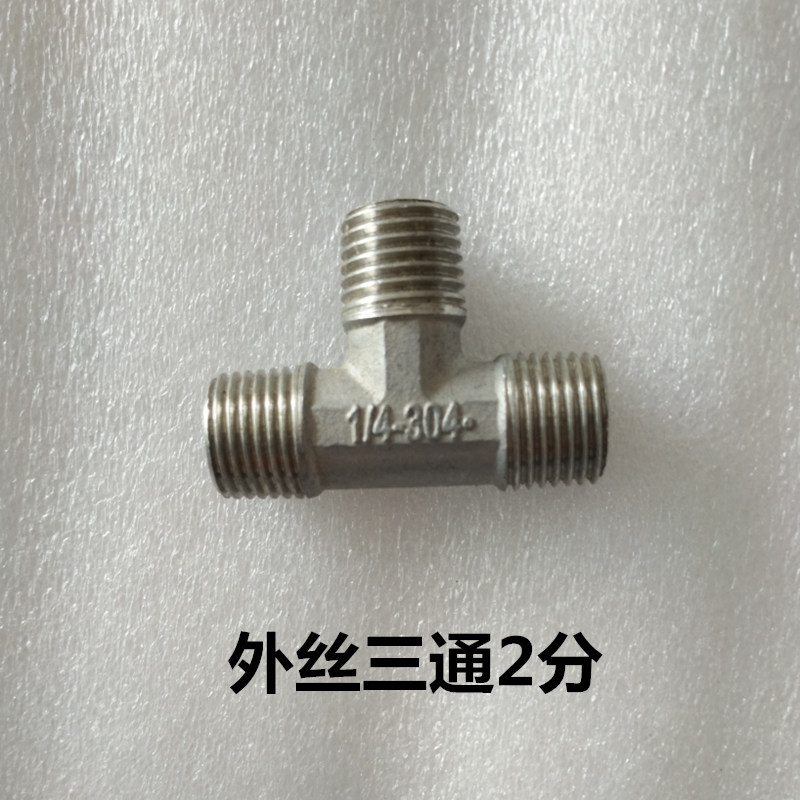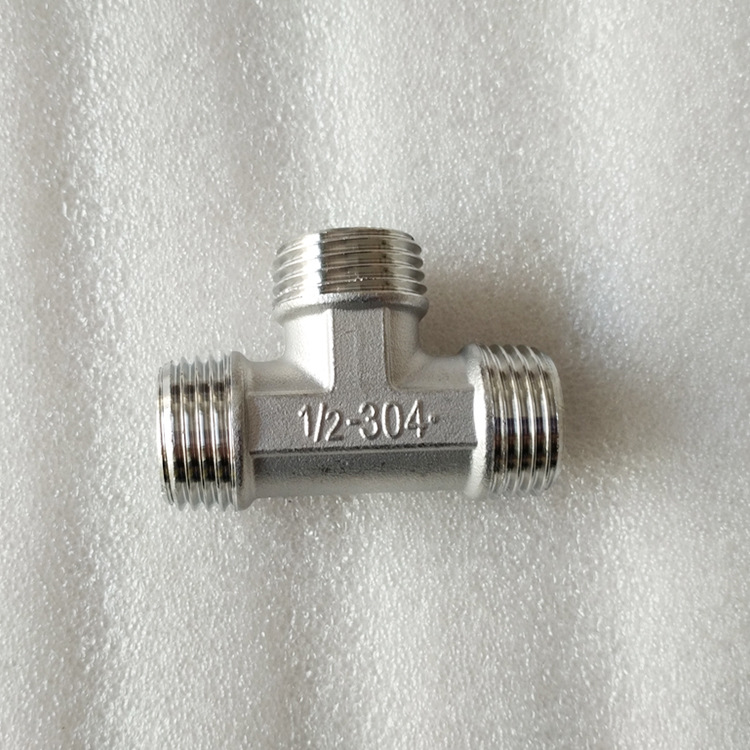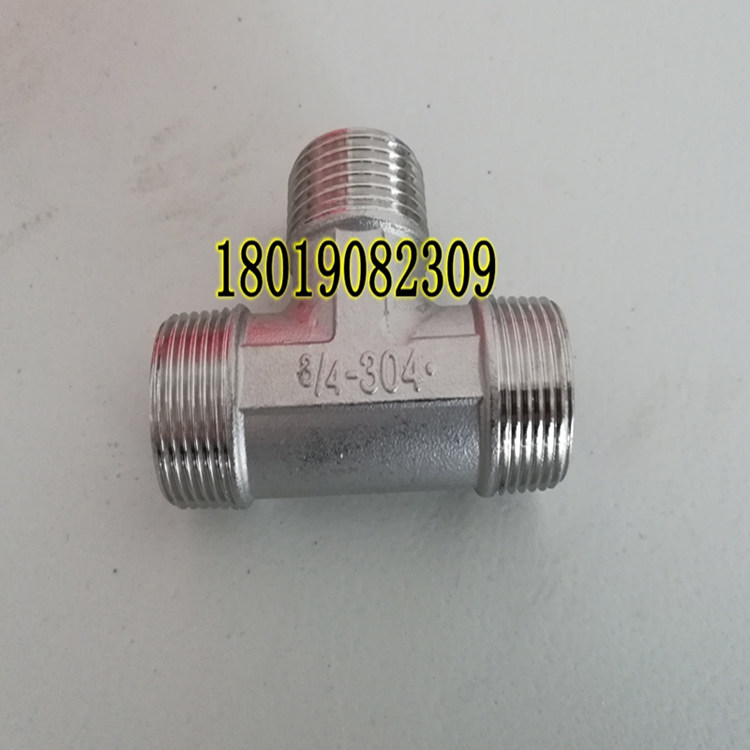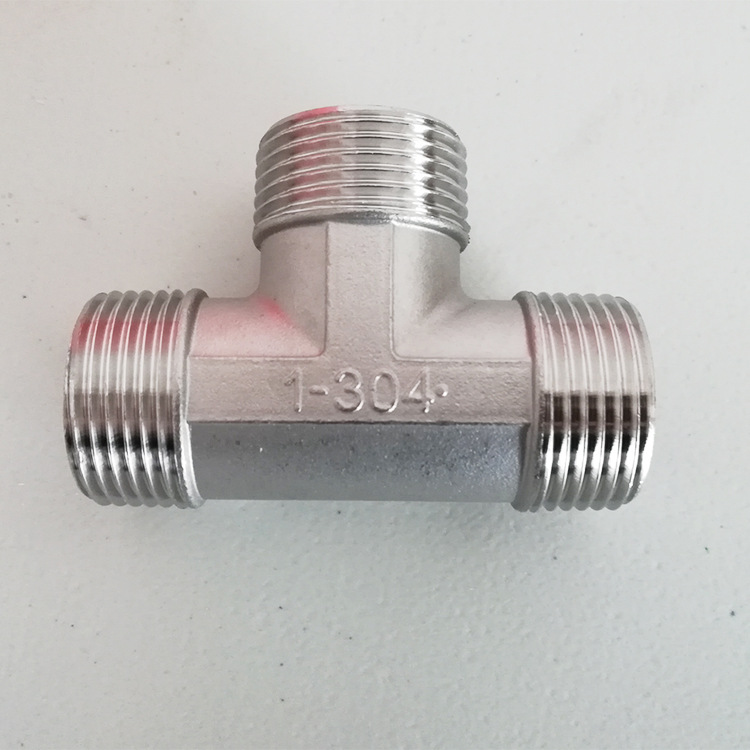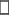手机版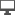电脑版